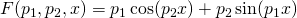## Simple nonlinear least squares curve fitting in Julia

December 6th, 2013 | Categories: Julia, programming | Tags:

A question I get asked a lot is ‘How can I do nonlinear least squares curve fitting in X?’ where X might be MATLAB, Mathematica or a whole host of alternatives.  Since this is such a common query, I thought I’d write up how to do it for a very simple problem in several systems that I’m interested in

This is the Julia version. For other versions,see the list below

The problem

xdata = -2,-1.64,-1.33,-0.7,0,0.45,1.2,1.64,2.32,2.9
ydata = 0.699369,0.700462,0.695354,1.03905,1.97389,2.41143,1.91091,0.919576,-0.730975,-1.42001

and you’d like to fit the functionusing nonlinear least squares.  You’re starting guesses for the parameters are p1=1 and P2=0.2

For now, we are primarily interested in the following results:

• The fit parameters
• Sum of squared residuals

Future updates of these posts will show how to get other results such as confidence intervals. Let me know what you are most interested in.

Julia solution using Optim.jl

Optim.jl is a free Julia package that contains a suite of optimisation routines written in pure Julia.  If you haven’t done so already, you’ll need to install the Optim package

Pkg.add("Optim")

The solution is almost identical to the example given in the curve fitting demo of the Optim.jl readme file:

using Optim

model(xdata,p) = p*cos(p*xdata)+p*sin(p*xdata)

xdata = [-2,-1.64,-1.33,-0.7,0,0.45,1.2,1.64,2.32,2.9]
ydata = [0.699369,0.700462,0.695354,1.03905,1.97389,2.41143,1.91091,0.919576,-0.730975,-1.42001]

beta, r, J = curve_fit(model, xdata, ydata, [1.0, 0.2])
# beta = best fit parameters
# r = vector of residuals
# J = estimated Jacobian at solution

@printf("Best fit parameters are: %f and %f",beta,beta)
@printf("The sum of squares of residuals is %f",sum(r.^2.0))

The result is

Best fit parameters are: 1.881851 and 0.700230
@printf("The sum of squares of residuals is %f",sum(r.^2.0))

Notes

I used Julia version 0.2 on 64bit Windows 7 to run the code in this post

1. Dear Mike Croucher,

I just wanted to let you know, that if you are interested in updating this post, the functionality is now in the LsqFit.jl package, instead of Optim. LsqFit still uses the Levenberg-Marquadt algorithm in Optim.jl, though. The curve_fit also returns results slightly different.

using LsqFit

model(xdata,p) = p*cos(p*xdata)+p*sin(p*xdata)

xdata = [-2,-1.64,-1.33,-0.7,0,0.45,1.2,1.64,2.32,2.9]
ydata = [0.699369,0.700462,0.695354,1.03905,1.97389,2.41143,1.91091,0.919576,-0.730975,-1.42001]

fit = curve_fit(model, xdata, ydata, [1.0, 0.2])

beta = fit.param # best fit parameters
r = fit.resid # vector of residuals
J = fit.jacobian # estimated Jacobian at solution

@printf(“Best fit parameters are: %f and %f”,beta,beta)
@printf(“The sum of squares of residuals is %f”,sum(r.^2.0))